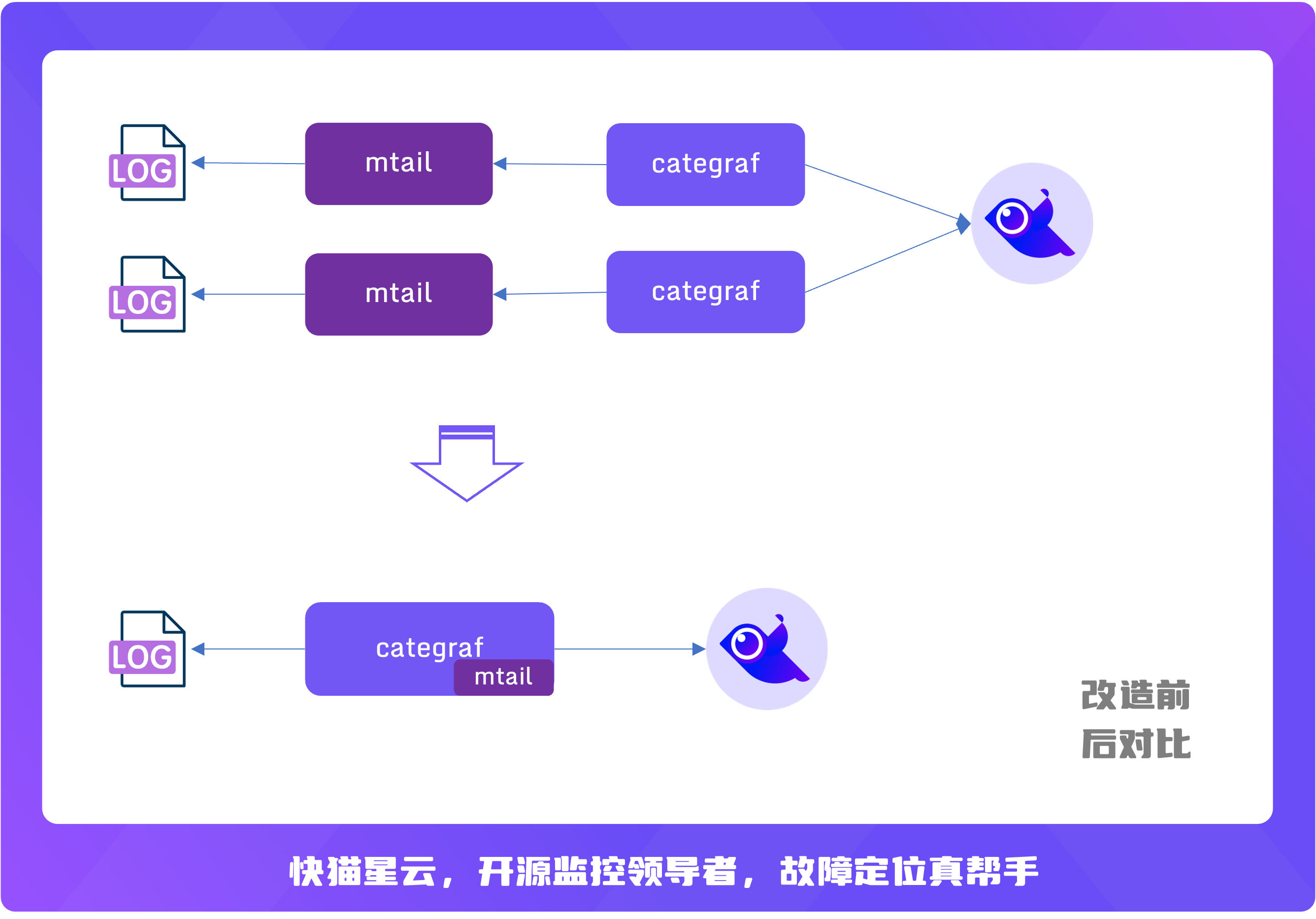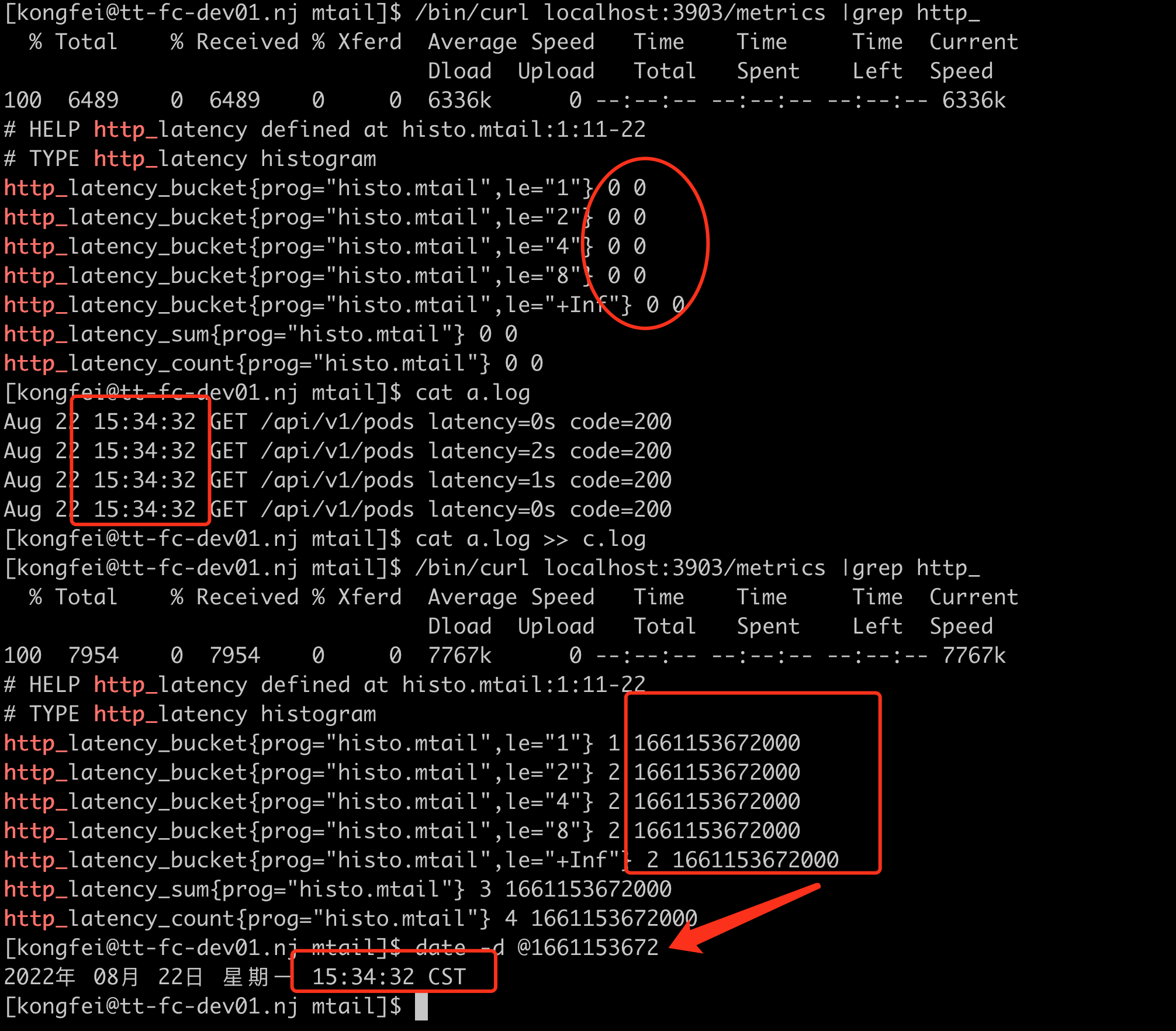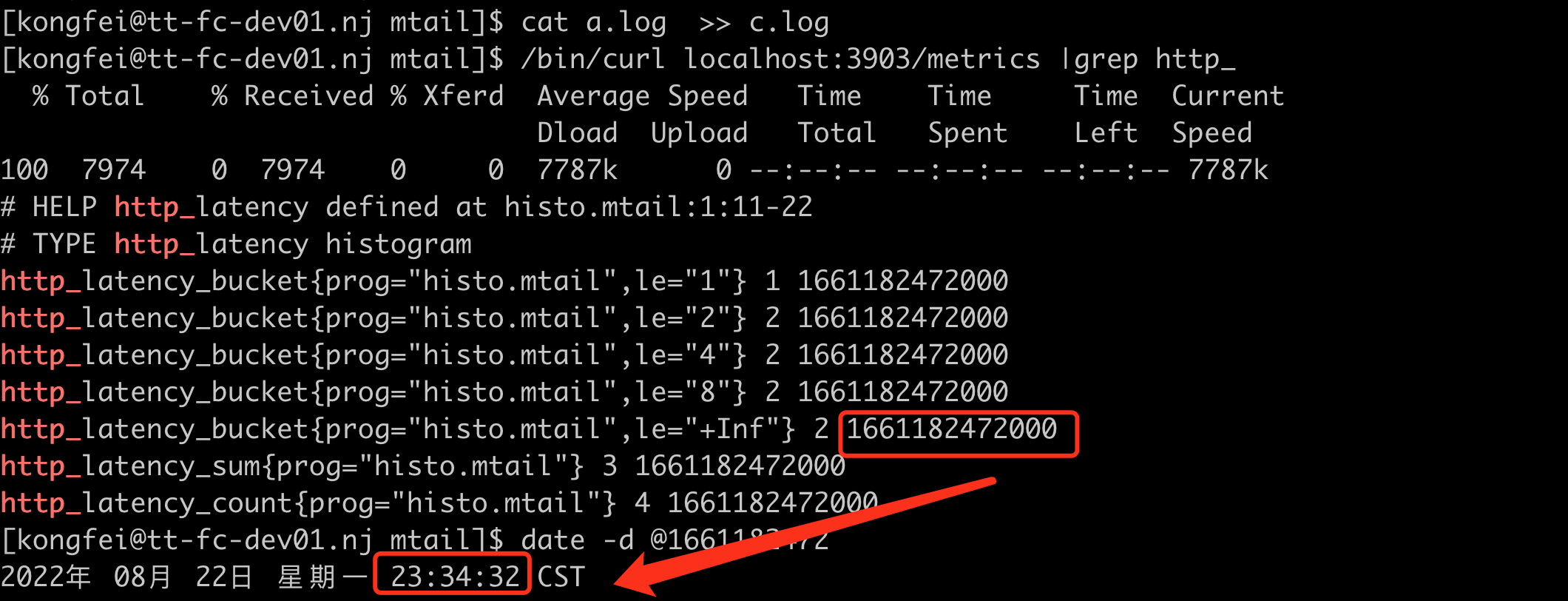## 从应用日志中提取监控metrics

mtail 是谷歌开源的一款从应用日志中提取 metrics 的工具。categraf 将 mtail 作为一个插件集成了进来，并且兼容 mtail 的语法，简化了部署。原本 mtail 和日志文件之间是一对一的关系，即一般都是一个 mtail 进程处理一类日志，但是对于很高配的物理机，上面会部署很多不同的服务，每个服务进程都对应一个 mtail，此时机器上会出现特别多的 mtail 进程，较难维护。把 mtail 集成到 categraf 之后，复用 categraf 的多实例插件机制，可以做到一台机器上只有一个 categraf 进程即可读取解析多个服务的日志。示例图如下：## 插件启动

### 输入

logs参数指定要处理的日志源, 支持模糊匹配, 支持多个log文件。

### 处理规则

`progs`指定具体的规则文件目录(或文件)

## 处理规则与语法

### 处理流程

``````for line in lines:
for regex in regexes:
if match:
do something
``````

### 语法

``````exported variable

pattern {
action statements
}

def decorator {
pattern and action statements
}
``````

#### 定义指标名称

``````counter lines
/INFO.*/ {
lines++
}
``````

``````counter lines_total as "line-count"
``````

#### 匹配与计算(pattern/action)

``````PATTERN {
ACTION
}

// examples:

/foo/ {
ACTION1
}

variable > 0 {
ACTION2
}

/foo/ && variable > 0 {
ACTION3
}
``````

``````const PREFIX /^\w+\W+\d+ /

PREFIX {
ACTION1
}

PREFIX + /foo/ {
ACTION2
}
``````

#### 关系运算符

• `<` 小于 `<=` 小于等于
• `>` 大于 `>=` 大于等于
• `==` 相等 `!=` 不等
• `=~` 匹配(模糊) `!~` 不匹配(模糊)
• `||` 逻辑或 `&&` 逻辑与 `!` 逻辑非

#### 数学运算符

• `|` 按位或
• `&` 按位与
• `^` 按位异或
• `+ - * /` 四则运算
• `<<` 按位左移
• `>>` 按位右移
• `**` 指数运算
• `=` 赋值
• `++` 自增运算
• `--` 自减运算
• `+=` 加且赋值

#### 支持else与otherwise

``````/foo/ {
ACTION1
} else {
ACTION2
}
``````

``````/foo/ {
/foo1/ {
ACTION1
}
/foo2/ {
ACTION2
}
otherwise {
ACTION3
}
}
``````

``````/(?P<operation>\S+) (\S+) \[\S+\] (\S+) \(\S*\) \S+ (?P<bytes>\d+)/ {
bytes_total[\$operation][\$3] += \$bytes
}
``````

``````# test.mtail
# 定义常量label env
hidden text env
# 给label 赋值 这样定义是global范围;
# 局部添加，则在对应的condition中添加
env="production"
counter line_total by logfile,env
/^(?P<date>\w+\s+\d+\s+\d+:\d+:\d+)/ {
line_total[getfilename()][env]++
}
``````

``````# metrics
line_total{env="production",logfile="/path/to/xxxx.log",prog="test.mtail"} 4 1661165941788
``````

``````# 日志内容
192.168.0.1 GET /foo
192.168.0.2 GET /bar
192.168.0.1 POST /bar
``````
``````# test.mtail
counter my_http_requests_total by log_file, verb
/^/ +
/(?P<host>[0-9A-Za-z\.:-]+) / +
/(?P<verb>[A-Z]+) / +
/(?P<URI>\S+).*/ +
/\$/ {
my_http_requests_total[getfilename()][\$verb]++
}
``````
``````# metrics
my_http_requests_total{logfile="xxx.log",verb="GET",prog="test.mtail"} 4242
my_http_requests_total{logfile="xxx.log",verb="POST",prog="test.mtail"} 42
``````

``````/(?P<x>\d+)/ && \$x > 1 {
nonzero_positives++
}
``````

#### 时间处理

``````http_latency_bucket{prog="histo.mtail",le="1"} 0
http_latency_bucket{prog="histo.mtail",le="2"} 0
http_latency_bucket{prog="histo.mtail",le="4"} 0
http_latency_bucket{prog="histo.mtail",le="8"} 0
http_latency_bucket{prog="histo.mtail",le="+Inf"} 0
http_latency_sum{prog="histo.mtail"} 0
http_latency_count{prog="histo.mtail"} 0
``````

``````http_latency_bucket{prog="histo.mtail",le="1"} 1 1661152917471
http_latency_bucket{prog="histo.mtail",le="2"} 2 1661152917471
http_latency_bucket{prog="histo.mtail",le="4"} 2 1661152917471
http_latency_bucket{prog="histo.mtail",le="8"} 2 1661152917471
http_latency_bucket{prog="histo.mtail",le="+Inf"} 2 1661152917471
http_latency_sum{prog="histo.mtail"} 3 1661152917471
http_latency_count{prog="histo.mtail"} 4 1661152917471
``````

``````Aug 22 15:28:32 GET /api/v1/pods latency=2s code=200
Aug 22 15:28:32 GET /api/v1/pods latency=1s code=200
Aug 22 15:28:32 GET /api/v1/pods latency=0s code=200
``````
``````histogram http_latency buckets 1, 2, 4, 8
/^(?P<date>\w+\s+\d+\s+\d+:\d+:\d+)/ {
strptime(\$date, "Jan 02 15:04:05")
/latency=(?P<latency>\d+)/ {
http_latency=\$latency
}
}
``````## 关于作者FlashcatFlashduty